Latest Banking jobs   »

# Quantitative Aptitude Quiz For LIC ADO Prelims 2023 -4th March

Q1. The ratio of the length and the breadth of a rectangular plot is 6 : 5 and the ratio of numerical value of perimeter and the area of this plot is 2 : 15. Find the perimeter of a square whose numerical value of its area is equal to numerical value of the perimeter of the rectangle?
(a) 40 cm
(b) 36 cm
(c) 44 cm
(d) 48 cm
(e) 52 cm

Q2. The cost price of a pencil is Rs 8 less than that of pen. When the pen is sold at 50% profit and pencil is sold at 150% profit it is found that the selling price of both is same. Find the cost price of pen.
(a) Rs. 20
(b) Rs. 24
(c) Rs. 25
(d) Rs. 16
(e) Rs. 15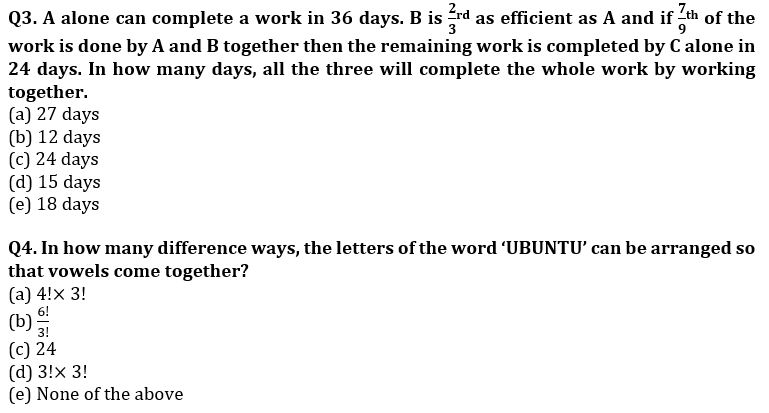Q5. If X : Y=2 : 3 & Y : Z=4 : 5 and average of X, Y & Z is 105 then find X+Z-Y?
(a) 87
(b) 90
(c) 105
(d) 96
(e) None of these

Direction (6-10): Table shows total number of students in 11th class of 5 different schools [A, B, C, D and E] and ratio of number of students in Art, Commerce and Science respectively.
Students study only the above given subjects in the class of each school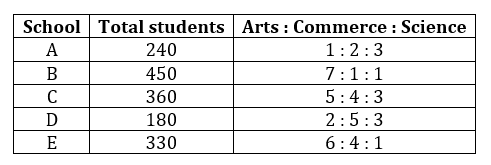Q6. What is total number of Science students in school A, B and C together?
(a) 240
(b) 260
(c) 290
(d) 220
(e) 310

Q7. What is difference between highest number of Art students in any school and lowest number of Commerce students in any school.
(a) 130
(b) 100
(c) 270
(d) 300
(e) 320

Q8. If it is known that, 20% of student got failed in class 10th of school C & out of those who passed 10% have left the school. Find the total number of students that was in class 10th in school C, given that no new student joins the school in 11th class.
(a) 500
(b) 400
(c) 480
(d) 540
(e) None of these

Q9. If ratio of Medical to Maths students in both school A and school E is 7: 8 then what is the difference between medical students of school A and maths students of school E? (Science branch of each school has only medical and maths students)
(a) 42
(b) 48
(c) 40
(d) 50
(e) None of these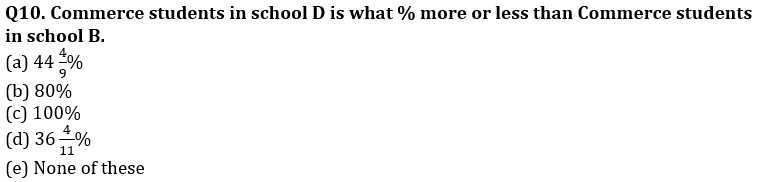Directions (11-14): In the following two equations questions numbered (I) and (II) are given. You have to solve both equations and Give answer
(a) If x > y
(b) If x ≥ y
(c) If y > x
(d) If y ≥ x
(e) If x = y or no relation can be established

Q11. I. x² – 8x + 15 = 0
II. 2y² – 7y + 5 = 0

Q12. I. 2x² + x – 28 = 0
II. 2y² – 23y + 56 = 0

Q13. I. 2x² – 7x – 60 = 0
II. 3y² + 13y + 4 = 0

Q14. I. x² – 17x – 84 = 0
II. y² + 4y – 117 = 0

Q15. In a party each person shakes hands exactly once with each of the others and total number of handshakes is 78. Then find the number of persons?
(a) 12
(b) 11
(c) 13
(d) 15
(e) 18

Solutions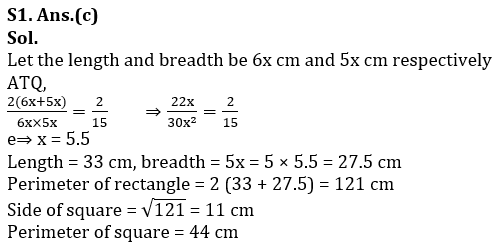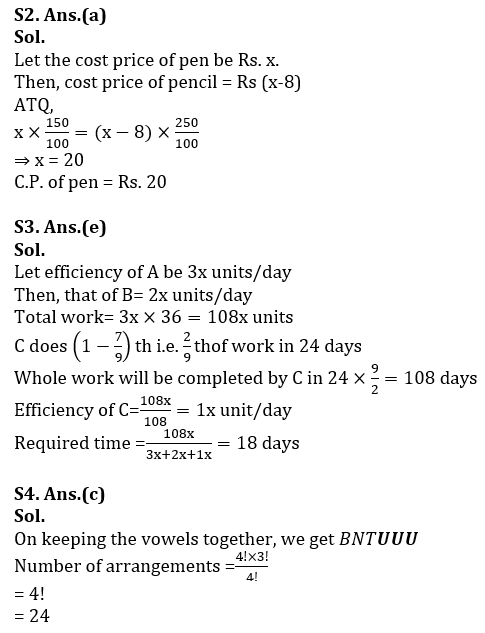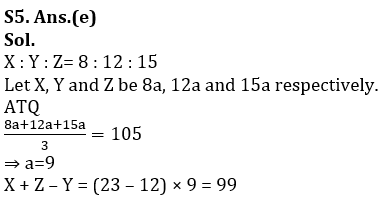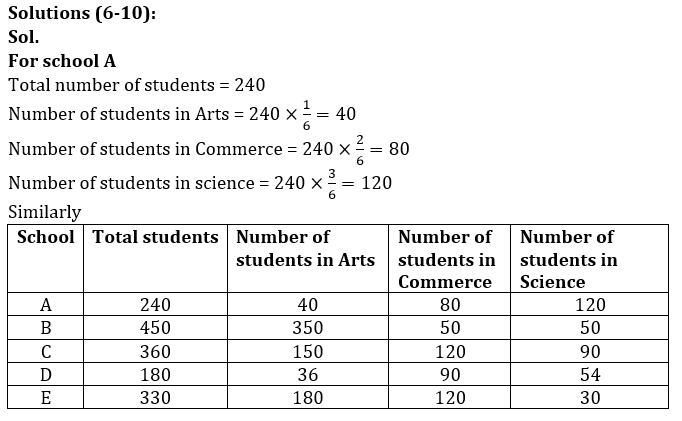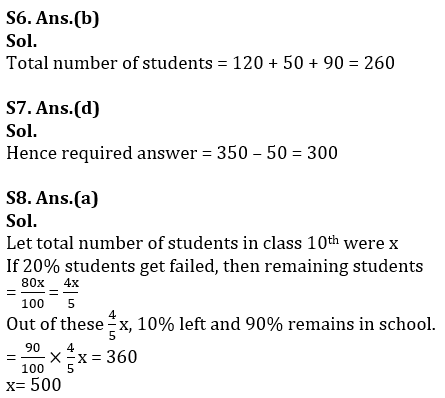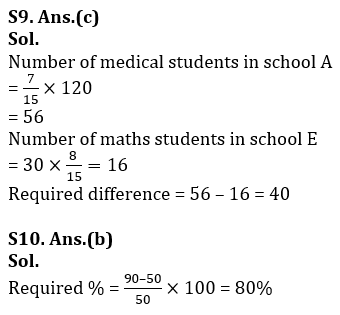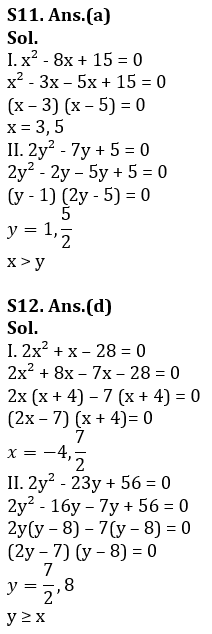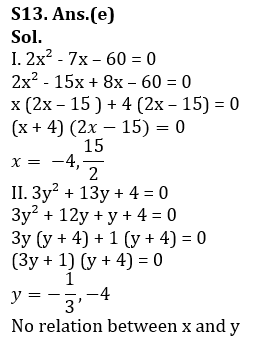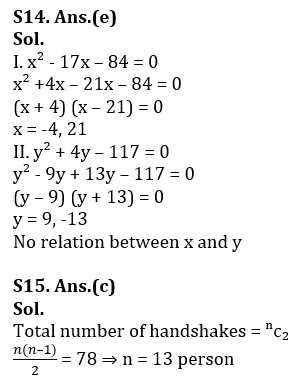.

## FAQs

### When will the LIC AAO Prelims Exam 2023 be conducted?

The LIC AAO Prelims Exam 2023 will be conducted on 17th & 20th February 2023.

#### Congratulations!Union Budget 2023-24: Free PDF